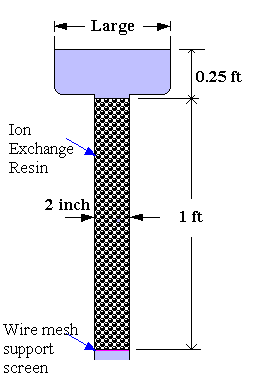### Flow Rate of Water through Ion-Exchange Column

Figure shows a water softener in which water trickles by gravity over a bed of spherical ion-exchange resin particles, each 0.05 inch in diameter. The bed has a porosity of 0.33. Calculate the volumetric flow rate of water.Calculations:

Data:

μ = 1 cp = 1 x 6.72x10-4 lb/(ft.sec)

ε = 0.33

ρ = 62.3 lb/ft3

ΔL = 1 ft

g = 32.2 ft/sec2

Formula:

Applying Bernoulli's equation from the top surface of the fluid to the outlet of the packed bed and ignoring the kinetic-energy term and the pressure drop through the support screen, which are both small, we find

g(ΔL) = hf

hf = Δp/ρ

For laminar flow, (Blake-Kozeny Equation)

$$\displaystyle \frac{\Delta p}{\rho} = 150\frac{V_s\mu(1-\varepsilon)^2\Delta L}{D_p^2\varepsilon^3\rho}$$

Calculations:

Therefore, Vs = 32.2 x 1.25 x (0.05/12)2 x 0.333 x 62.3 / ( 150 x (1 x 6.72x10-4) x (1 - 0.33)2 x 1)

= 0.035 ft/sec = 0.011 m/sec.

Q = AVs = (2/12)2 x (π/4) x 0.035 = 0.00075 ft3/sec = 21 cm3/sec

Before accepting this as the correct solution, we check the NRepm.

NRepm= (0.05/12) x 0.035 x 62.3 / (1 x 6.72x10-4 x (1 - 0.33) ) = 20.2

This is slightly above the value of 10 (up to which the Blake-Kozeny Equation can be used), for which we can safely use without appreciable error.